SYLLABUS  Previous: 5.3 Methods for bonds  Up: 5.3 Methods for bonds  Next: 5.3.2 Extensions for derivatives

5.3.1 The Vasicek model for a bond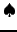[ SLIDE variational form - implicit time - FEM - code - run || VIDEO modem ( 1/ 2 ) - LAN ( 1/ 2 ) - DSL ( 1/ 2)]

The finite element method offers considerable advantages in terms of flexibility and robustness at the expense of a slightly more complicated formulation. For example, the FEM method does not suffer from the numerical instability that limits the time stepping in finite difference schemes, it converges very quickly in comparison with the Monte-Carlo method using only few driving factors and the next chapter will show how the formulation can easily be extended to deal with the American exercise style. Here we use the Vasicek equation (3.5#eq.6) only to illustrate one implementation and justify the VMARKET solution for the price of a bond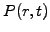as a function of the spot rate and time. More details about the finite element method and its formulation can be found on-line.
Since the random increments of interest rates are normally distributed in the Vasicek model, there is no real advantage to transform the problem into normalized variables. We therefore start directly from the partial differential equation (3.5#eq.6)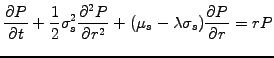Multiply by an arbitrary test function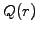, integrate over the domain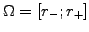where the solution is sought and formulate a variational principle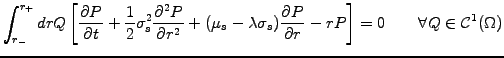(5.3.1#eq.1)

It turns out that this variational problem is equivalent to the original equation provided that the equation is satisfied for all the test functions that are sufficiently general''. Galerkin's method of choosing the same functional space as the solution is an excellent starting point: here it is sufficient to keep piecewise linear function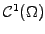. Indeed, after integration by parts of the second order term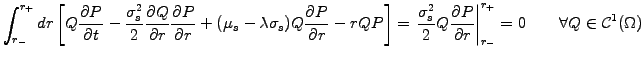(5.3.1#eq.2)

only first order derivatives remain, which can be evaluated using linear functions. The surface term that has been produced is used to impose so-called natural boundary conditions: the contribution from the upper boundary vanishes if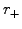is large enough, since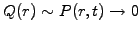for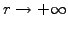when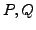are chosen from the same functional space. Essential boundary conditions will be imposed on the lower boundary to normalized the discount function where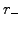is small, so that the surface term can here simply be neglected. Now discretize the time into small steps using a backward difference for the first term and a partly implicit evaluation for the rest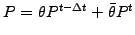where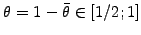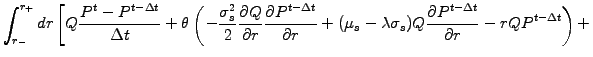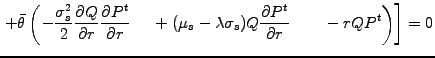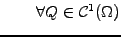(5.3.1#eq.3)

Discretize the interest rates, decomposing the solution into the weighted sum of finite elements roof-top functions suggested in (5.3.1#fig.1). Reassemble dependencies on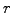into overlap integrals of the form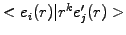, which can be calculated analytically for a homogeneous mesh. Assuming a constant volatility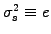in (3.5#eq.7) and drift of the form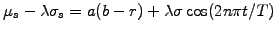, the second last term is conveniently re-written using the coefficient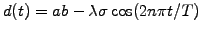as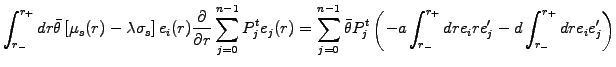Multiply by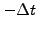and write all the unknown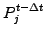as a function of the known values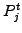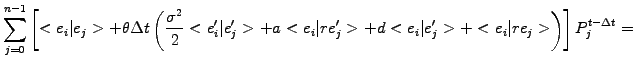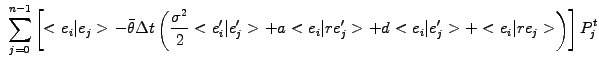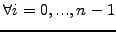(5.3.1#eq.4)

To complete the formulation, the problem has to be supplemented with a terminal condition: from the definition of the discount function (2.2.2#eq.1) this is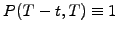when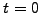. Boundary conditions have to be justified from no-arbitrage considerations: for simplicity, the yield in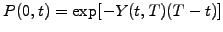(2.2.2#eq.1) is here somewhat artificially associated with the spot rate and forced to zero with the Dirichlet condition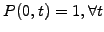. A similar reasoning justifies the Neuman condition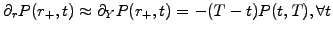and is here implemented for a homogeneous grid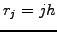using the second order finite difference approximation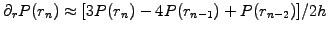.
Because of the finite extension of finite element roof-top functions overlapping only with the nearest neighbors, the linear system of equations (5.3.1#eq.4) can be cast into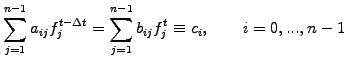(5.3.1#eq.5)

The matrix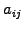is tridiagonal of the form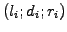, except the last row, where an element created by the Neuman condition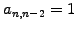has to be eliminated by hand (row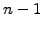minus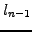times row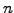) to preserve the structure of the matrix(5.3.1#eq.6)

After substituting the value for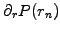and replacing the last equation by (5.3.1#eq.6), the linear system is solved using standard LU factorization.

Quants: linear Galerkin finite element (FEM) discretization.
Decompose the solution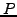into a superposition of finite element roof-top functions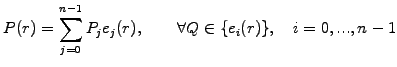(5.3.1#eq.7)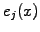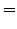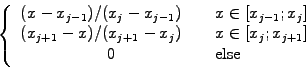(5.3.1#eq.8)

choosing the same elements for the test function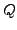to create as many equations as unknowns.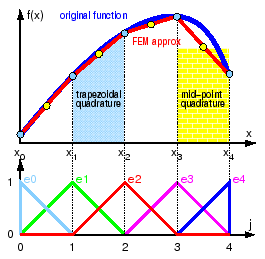Only the nearest neighbors contribute to the overlap integrals; these can be evaluated with a combination of the trapezoidal and the mid-point rule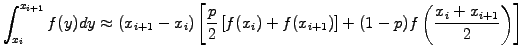(5.3.1#eq.9)

where a suitable choice of the tunable integration parameter reproduces piecewise constant (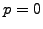) or linear FEM approximations (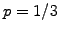) or the Crank-Nicholson method (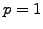). For a homogeneous mesh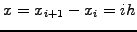, the overlap integrals can be calculated analytically and the finite contributions yield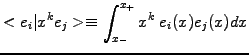(5.3.1#eq.10)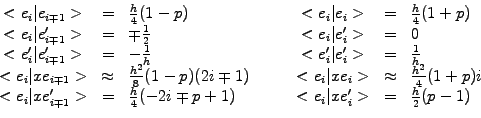Despite the rather sophisticated derivation, this finally yields a very elegant scheme that has been implemented in the VMARKET class FEMSolution.java as
double twopi    = 8.*Math.atan(1.);
double runTime  = runData.getParamValue("RunTime");
double timeStep = runData.getParamValue("TimeStep");
double sigma    = runData.getParamValue("Volatility");
double theta    = runData.getParamValue("TimeTheta");
double lambda   = runData.getParamValue("MktPriceRsk");
double t        = 1.-time/runTime;               // normalized time
double hump     = 1.7*(t-t*t*t*t*t*t);           // volatility shaping
double ca       = runData.getParamValue(runData.meanRevVeloNm);
double cb       = runData.getParamValue(runData.meanRevTargNm);
double cycles   = runData.getParamValue(runData.userDoubleNm);
double cd       = ca*cb-lambda*sigma*Math.cos(twopi*cycles*t);
double ce       = sigma*hump; ce=ce*ce;
//--- CONSTRUCT MATRICES
BandMatrix a = new BandMatrix(3, f.length);      // Linear problem
BandMatrix b = new BandMatrix(3, f.length);      //  a*fp=b*f=c
double[]   c = new double[f.length];
double h,h0,h0o,h1,h1m,h1p,h2,h2o;               //  independent of i
double t0,t0m,t0p,t1,t1m,t1p;                    //  depending on i
h= dx;
h0o= 0.25*h*(1-tune);               h0= 0.5*h*(1+tune);
h1m=-0.5; h1p=-h1m;                 h1= 0.;
h2o=-1./h;                          h2= 2./h;
for (int i=0; i<=n; i++) {
t0m=h*h*0.125*(1-tune)*(2*i-1);
t0p=h*h*0.125*(1-tune)*(2*i+1);   t0=h*h*0.5*i*(tune+1);
t1m=h*h*0.25*(-2*i-tune+1);
t1p=h*h*0.25*(-2*i+tune+1);       t1=h*h*0.5*(tune-1);
a.setL(i,h0o + theta   *timeStep*(t0m +0.5*ce*h2o +ca*t1m +cd*h1m));
a.setD(i,h0  + theta   *timeStep*(t0  +0.5*ce*h2  +ca*t1  +cd*h1 ));
a.setR(i,h0o + theta   *timeStep*(t0p +0.5*ce*h2o +ca*t1p +cd*h1p));
b.setL(i,h0o +(theta-1)*timeStep*(t0m +0.5*ce*h2o +ca*t1m +cd*h1m));
b.setD(i,h0  +(theta-1)*timeStep*(t0  +0.5*ce*h2  +ca*t1  +cd*h1 ));
b.setR(i,h0o +(theta-1)*timeStep*(t0p +0.5*ce*h2o +ca*t1p +cd*h1p));
}
c=b.dot(f);
double dPdy0, dPdyn, c0, cn;                     //--- BC
a.setL(0, 0.);a.setD(0, 1.);a.setR(0, 0.);c=1.;//left: Dirichlet
double a1n= a.getD(n-1) +4.*a.getL(n-1);         // right: Neuman
double ann= a.getR(n-1) -3.*a.getL(n-1);         //   O(h^2)
dPdyn=-time*f[n]; cn=c[n-1]-2*dx*a.getL(n-1)*dPdyn;
a.setL(n,a1n);a.setD(n,ann);a.setR(n, 0.);c[n]=cn;
fp=a.solve3(c);                                  //--- SOLVE
for (int i=0; i<=n; i++) {                       //--- PLOT
gp[i]=-Math.log(fp[i])/time;                   // yield(r)
if (time<=timeStep) f0[i]=0.;
}
int i=(int)((time/runTime*n));                   // yield(t)
f0[i]=gp[n/4];
Two band matrices a,b and a vector c are created to first assemble the linear problem (5.3.1#eq.5) using the commands of the form a.setL(j,*): they define matrix elements in row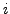either to the Left, Right or on the Diagonal of the matrices a,b. The right hand side vector is calculated with a product between the matrix b and the discount function f that is known from the previous time step. The solution fp is computed using LU factorization and the yield is defined from the discount function (2.2.2#eq.1) ready for plotting.
The VMARKET applet below shows the result obtained for a weakly implicit scheme (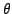or TimeTheta=0.55) and a tunable integration parameter (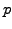or TuneQuad=1.), which is equivalent to the popular Crank-Nicholson method used by the finite differences afficinados; to be financially meaningful, the solution has of course to be independent of the numerical method.

implicit finite differences but results in the same computational cost. The additional flexibility provided by a finite elements formulation is such that the Crank-Nicholson scheme should in fact be of little more than historical interest.

SYLLABUS  Previous: 5.3 Methods for bonds  Up: 5.3 Methods for bonds  Next: 5.3.2 Extensions for derivatives